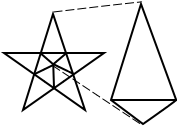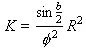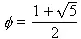On-line GuidesAll GuideseBook StoreiOS / AndroidLinux for BeginnersOffice ProductivityLinux InstallationLinux SecurityLinux UtilitiesLinux VirtualizationLinux KernelSystem/Network AdminProgrammingScripting LanguagesDevelopment ToolsWeb DevelopmentGUI Toolkits/DesktopDatabasesMail SystemsopenSolarisEclipse DocumentationTechotopia.comVirtuatopia.comHow To GuidesVirtualizationGeneral System AdminLinux SecurityLinux FilesystemsWeb ServersGraphics & DesktopPC HardwareWindowsProblem SolutionsPrivacy Policy## Chapter 37. Areas of the Flag

From Robert Banks, Slicing Pizzas, Racing Turtles, and Further Adventures in Applied Mathematics [Banks02].

This project is simple: it does not use loops, if-statements or any data structures. This exercise focuses on expressions and assignment statements.

Facts about the American flag. The standard dimension is the width (or hoist). This is the basic unit that all others are multiplied by. A flag that is 30 inches wide will simply have 30 inches multiplied by all of the other measurements.

• Width (or hoist ) Wf=1.0
• Length (or fly ) Lf=1.9 × Wf
• Width of union (or canton ) Wu=7/13 × Wf
• Length of union Lu=0.76 × Wf
• Radius of a star R=0.0308 × Wf

These are other facts; they are counts, not measurements.

• Number of red stripes Sr=7
• Number of white stripes Sw=6
• Number white stars Ns=50
• Width of a stripe Ws=1/(Sr+Sw) × Wf

Red Area. There are 4 red stripes which abut the blue field and 3 red stripes run the full length of the flag.

We can compute the area of the red, since it is 4 short rectangles and 3 long rectangles. The short rectangle areas are the width of a stripe (Ws) times the whole length (length of the fly, Lf) less the width of the blue union (Wu). The long rectangle areas are simply the width of the stripe (Ws) times the length of the fly (Lf).

Red = 4×Ws×(Lf-Lu) + 3×Ws×(Lf).

White Area. There are 3 white stripes which abut the blue field, 3 whie stripes run the full length of the flag, plus there are the 50 stars.

We can compute the basic area of the white using a similar analysis as area of the red, and adding in the areas of the stars, 50 S . We'll return to the area of the stars, 50 S , last.

White = 3×Ws×(Lf-Lu) + 3×Ws×(Lf) + 50S.

Blue Area. The blue area is the area of the union, less the area of the stars, 50 S .

Blue = (Lu-Wu) - 50S.

Area of the Stars. A 5-sided star (pentagram) can be analyzed as 5 kites of 2 triangles. The area of each kite, K , is computed as follows.

Figure 37.1. Kite and StarThe inner angles of all five kites fill the inside of the pentagram, and the angles must sum to 360°, therefore each inner angle is 72°. Angles of a triangle sum to 180°, therefore the lower triangle has two side angles of (180°-72°)/2 = 54°.

We see some straight lines that contain an outer triangle and two inner triangles. We know the inner triangles add to 108°, a straight line is 180° so the outer triangle has two 72° corners and a 36° peak. The area of the two triangles can be computed as

a=36°, b=72°

Equation 37.1. Area of a triangle, version 1Equation 37.2. Area of a triangle, version 2Note that the math library `sin` and `cos` functions operate in radians, not degrees. The conversion rule is π radians = 180 degrees. Therefore, we often see something like `sin `( ``` a ×π/180 ``` ) to convert `a` degrees to radians.

The Golden Ratio is(about 1.61803).

The total area of a star is

S=5×K.

Given a specific width of a flag (in feet), we can compute the actual areas of each color.

Check Your Results. Blue is 18.73% of the total area.Published under the terms of the Open Publication License Design by Interspire